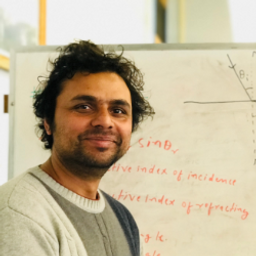## no answer available

#### Topics

Electromagnetic Waves

Wave Optics

### Discussion

You must be signed in to discuss.
##### Top Physics 103 Educators##### Andy C.

University of Michigan - Ann Arbor##### Marshall S.

University of Washington##### Jared E.

University of Winnipeg### Video Transcript

in this problem, we have the length of the microscope. That is the distance between the objective and I piss is 20. Senator, The lateral magnification of the objective is 10 and I'm using the negative side because the object is upside down, the image of the object is upside down, and the overall magnification of the microscope is 100 and 15. And I'm using negative sand just as the same reason for the lateral magnification. Okay, so in part A to find the angular magnification of the eyepiece. What we're gonna do is we're gonna use the definition for the lateral magnification, which is MM equals m one dot e. So from here, M e, which is the magnification of the eyepiece, is going to be him over and one, and that's going to be a negative 115 over negative 10. And this is going to give me 11.5. So this is the angular magnification of the apis. So for part B, to find the focal length of the eyepiece, we basically just use M e. The definition of the angler magnification for the I piss is going to be 25 centimeter over focal length of the eyepiece. And from here, F E is going to be 25 centimeter over M E. And we know at me. So 25/11 0.5 and this will give me 2.17 centimeter. So this is the focal length of the eyepiece. Now this go to Parsi, in which we are trying to find the objective for collect. So what we're gonna do is we're gonna use the definition of the lateral magnification of the objective, which is gonna be negative over focal length of the objective. And the focal length of the objective is going to be negative. Hell over M one and negative. L is negative. 20 centimeter over negative 10. So this will give me two centimeter.University of Wisconsin - Milwaukee

#### Topics

Electromagnetic Waves

Wave Optics

##### Top Physics 103 Educators##### Andy C.

University of Michigan - Ann Arbor##### Marshall S.

University of Washington##### Jared E.

University of Winnipeg